Triangular well model

The triangular well model, proposed by Takeo Nagayima in one dimension , is given by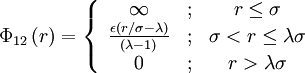$\Phi_{12}\left( r \right) = \left\{ \begin{array}{ccc} \infty & ; & r \leq \sigma \\ \frac{\epsilon (r/\sigma - \lambda)}{(\lambda -1)} & ; &\sigma < r \leq \lambda \sigma \\ 0 & ; & r > \lambda \sigma \end{array} \right.$

where$\Phi_{12}(r)$ is the intermolecular pair potential,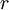$r$ is the distance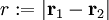$r := |\mathbf{r}_1 - \mathbf{r}_2|$,$\sigma$ is the hard diameter,$\epsilon$ is the well depth and λ > 1.

Virial coefficients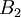$B_2$,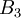$B_3$   and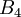$B_4$